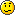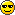# Set high and set low?

Junior MemberMember Posts: 20 ■□□□□□□□□□
For a quick example in using the above table/pattern, let us
figure out the IP range for the subnet indicated by:
172.16.100.32/28

First we notice that the network address is 172.16.100.32, so we
know that the subnet begins with this address. Second, we notice that the
bitmask of 28 indicates that the last 4 bits (32-28 ) are set low and 28
bits set high.

Im just inquiring about the "set low" and "set high" stuff. what does that all mean? and is it needed for the Net+?:
CQ Services, Inc.
ISP, Repair, Web Hosting, Web Design, Online Advertising, & Computer Sales

This is one of the many (and one of the worse I've seen) different explanations of subnetting. Set low simply means they are 0... high is 1.

This is NOT part of Network+. Yes, subnet masks are part of Network+, but 'subnetting' refers to dividing subnets. It is an essential part of CCNA and several other Cisco exams, but for Network+ you only need to know the major subnets (/8 /16 /24).

If you do want to know how subnetting (borrowing host bits to create more network) and supernetting (vice versa) www.learntosubnet.com is still the best place to start.
• Junior Member Member Posts: 20 ■□□□□□□□□□
thanks budCQ Services, Inc.
ISP, Repair, Web Hosting, Web Design, Online Advertising, & Computer Sales
• Senior Member Member Posts: 542
Lemme see if I remember my subnetting....

/28 translates to 255.255.255.240. Subtract the custom (240) from 256 to get the range of IP's for each subnet, in this case 16. That means the first subnet will be... 172.16.100.0 to 172.16.100.15. The subnet they're currently asking about will be 172.16.100.32 to 172.16.100.47. Network ID ip is 172.16.100.32, broadcast is 172.16.100.47, usable host ID's are 172.16.100.33 through 172.16.100.46.

If that's wrong someone let me know= Marcus Drakonblayde
================
CCNP-O-Meter:
=[0%]==[25%]==[50%]==[75%]==[100%]
==[X]===[X]====[ ]=====[ ]====[ ]==
=CCNA==BSCI==BCMSN==BCRAN==CIT=
• Member Member Posts: 73 ■■□□□□□□□□
So... you need to be able to figure out the binary part of it?
Like in the above example. Or is this overkill for the Net+?

None of my study materials cove it at all. I'm using Sybex, Beachfront, and this site.:
She don't come, and I don't follow.
• Grumpy old bugger Member Posts: 2,068 ■■■□□□□□□□
Overkill - BUT always good to know.
www.supercross.com
FIM website of the year 2007
• Senior Member Member Posts: 1,017
you will need to know that stuff for cisco and microsoft exams, but for network+ all you need to know is the default subnets for the classes and the classless notation for the same.
A+ Moderator
• Senior Member Member Posts: 542
The easiest way to learn subnetting is to know the value of each of the places for the first 8 bits.

128 64 32 16 8 4 2 1

Say you've got

10110001

That tells me that the 128 bit is on, the 32 bit is on, the 16 bit is on and the 1 bit is on, so adding those three values together gives you 177. If you think in multiples of two, it becoems easy to translate binary on sight.

With a custom subnet mask (ie, not a standard class a (/, class b (/16), or class C (/24) subnet mask, the bits are turned on from left to right. There must always be at least two bits available for host addresses, so the biggest subnet mask you will ever see is 255.255.255.252.

Now it gets really confusing depending on what you want to break up. But essentially, the value of the furthest bit to the right turned on tells you the range of the subnet and the amount of subnets you'll have.

The first subnet and the last subnet are totally unusable. Why? They contain the network ID and the broadcast ID for the entire network. This is why subnetting can waste a whole lot of IP's.

Now, let's say I have a class B address of 160.12.0.0 and I give it a subnet mask of 255.255.224.0.

That tells me I have 19 bits turned on for the network ID, leaving me 13 bits for host addresses. I'm going to assume that 255 is the binary equivalent of 8 1's is obvious. For the 224, that means 3 of 8 bits are on,

11100000

Giving me the values of 128+64+32, which works out to 224.

Now there are two ways to determine the range of each subnect. Easiest way is to subtract the custom subnet from 256. 256 - 224 = 32. The other way is to check the value of the right most bit turned on. In this case, the third bit from the left is 32, so that checks. Your range also determines how many subnets you'll have. Divide 256 by your range and that will tell you how many possible subnets you have. In this case, it'll be 8 possible, 6 useable.

13 bits are left for host ID's. 13^2 = 8192. Two of these are not usable, the very first address is the network id for the subnet, and the very last is the broadcast for the subnet. So total number of useable hosts is 8190.

Remember what I said about subnetting being wasteful? The first and last subnets aren't useable, which means about 8200 ip's per subnet can be used. Just by breaking the network up, you'd be wasting 16,000 ip's.

To bring this all together...

Range = 32 (this is the range between subnets)
Hosts per subnet = 8190
Number of subnets = 6

The very first subnet would be 160.12.0.0 to 160.12.31.255. This subnet is not usable. Remember 0 is a valid digit, so 0 to 31 is a range of 32.

The first usable subnet is 160.12.32.0 to 160.12.63.255. Valid host IP's are from 160.12.32.1 to 160.12.63.254. The last subnet, also unuseable, is 160.12.224.0 to 160.12.255.255. The way to figure that out is that the beginning of the last subnetwill be the same as your custom host mask. That also tells you the easiest way to figure your last useable subnet... custom netmask - range. So it'd be 160.12.192.0 to 160.12.223.255.

You can also very quickly figure out the value of any subnet just by multiplying it by the range. Say I wanted to know the fifth useable subnet. 32 * 5 = 160. So the start IP of the 5th subnet would be 160.12.160.0 and would end with 160.12.191.255.

And no, this stuff isn't on Net+ hehe

Hope this helps somebodyIt's the condensed version of what I was taught

edit: corrected spelling error and accidentally said first two subnets were unuseable instead of first and last
= Marcus Drakonblayde
================
CCNP-O-Meter:
=[0%]==[25%]==[50%]==[75%]==[100%]
==[X]===[X]====[ ]=====[ ]====[ ]==
=CCNA==BSCI==BCMSN==BCRAN==CIT=
• Senior Member Member Posts: 288
Well, all I can say is bring on IPv6 and away goes subnetting!Cheeblie
• Senior Member Member Posts: 542
Not for awhile though. You've got an entire infrastructure built on ipv4, and that's not going to change overnight. And I'm sure ipv6 will bring it's own complications to the game. The more things change, the more they stay the same= Marcus Drakonblayde
================
CCNP-O-Meter:
=[0%]==[25%]==[50%]==[75%]==[100%]
==[X]===[X]====[ ]=====[ ]====[ ]==
=CCNA==BSCI==BCMSN==BCRAN==CIT=
• Grumpy old bugger Member Posts: 2,068 ■■■□□□□□□□
IPv6 aye .... when? is what I ask.

From what I hear it will not be coming to us anytime in the near future. A little issue about a certain brand router not being able to handle IPv6.
www.supercross.com
FIM website of the year 2007
The problem lies in the simple fact that you can't change everything all at once so there has to be a migration path, which means that for a long time we'll have both IPv4 and IPv6... and who wants that?